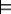### 5.4.1 Horn Clauses

The definite clause language does not allow a contradiction to be stated. However, a simple expansion of the language can allow proof by contradiction.

An integrity constraint is a clause of the form

false←a1∧...∧ak.

where the ai are atoms and false is a special atom that is false in all interpretations.

A Horn clause is either a definite clause or an integrity constraint. That is, a Horn clause has either false or a normal atom as its head.

Integrity constraints allow the system to prove that some conjunction of atoms is false in all models of a knowledge base - that is, to prove disjunctions of negations of atoms. Recall that ¬p is the negation of p, which is true in an interpretation when p is false in that interpretation, and p∨q is the disjunction of p and q, which is true in an interpretation if p is true or q is true or both are true in the interpretation. The integrity constraint false←a1∧...∧ak is logically equivalent to ¬a1∨...∨¬ak.

A Horn clause knowledge base can imply negations of atoms, as shown in Example 5.16.

Example 5.16: Consider the knowledge base KB1:
false←a∧b.
a←c.
b←c.

The atom c is false in all models of KB1. If c were true in model I of KB1, then a and b would both be true in I (otherwise I would not be a model of KB1). Because false is false in I and a and b are true in I, the first clause is false in I, a contradiction to I being a model of KB1. Thus, c is false in all models of KB1. This is expressed as

KB1¬c

which means that ¬c is true in all models of KB1, and so c is false in all models of KB1.

Although the language of Horn clauses does not allow disjunctions and negations to be input, disjunctions of negations of atoms can be derived, as Example 5.17 shows.

Example 5.17: Consider the knowledge base KB2:
false←a∧b.
a←c.
b←d.
b←e.

Either c is false or d is false in every model of KB2. If they were both true in some model I of KB2, both a and b would be true in I, so the first clause would be false in I, a contradiction to I being a model of KB2. Similarly, either c is false or e is false in every model of KB2. Thus,

 KB_2¬c∨ ¬d. KB_2¬c∨ ¬e.

A set of clauses is unsatisfiable if it has no models. A set of clauses is provably inconsistent with respect to a proof procedure if false can be derived from the clauses using that proof procedure. If a proof procedure is sound and complete, a set of clauses is provably inconsistent if and only if it is unsatisfiable.

It is always possible to find a model for a set of definite clauses. The interpretation with all atoms true is a model of any set of definite clauses. Thus, a definite-clause knowledge base is always satisfiable. However, a set of Horn clauses can be unsatisfiable.

Example 5.18: The set of clauses {a, false←a} is unsatisfiable. There is no interpretation that satisfies both clauses. Both a and false←a cannot be true in any interpretation.

Both the top-down and the bottom-up proof procedures can be used to prove inconsistency, by using false as the query. That is, a Horn clause knowledge base is inconsistent if and only if false can be derived.